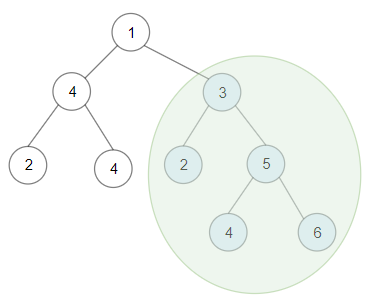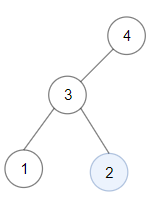1373. Maximum Sum BST in Binary Tree

Hard

Given a binary tree root, the task is to return the maximum sum of all keys of any sub-tree which is also a Binary Search Tree (BST).
Assume a BST is defined as follows:

• The left subtree of a node contains only nodes with keys less than the node’s key.
• The right subtree of a node contains only nodes with keys greater than the node’s key.
• Both the left and right subtrees must also be binary search trees.#### Example 1:

Input: root = [1,4,3,2,4,2,5,null,null,null,null,null,null,4,6]
Output: 20
Explanation: Maximum sum in a valid Binary search tree is obtained in root node with key equal to 3.#### Example 2:

Input: root = [4,3,null,1,2]
Output: 2
Explanation: Maximum sum in a valid Binary search tree is obtained in a single root node with key equal to 2.


#### Example 3:

Input: root = [-4,-2,-5]
Output: 0
Explanation: All values are negatives. Return an empty BST.


#### Example 4:

Input: root = [2,1,3]
Output: 6


#### Example 5:

Input: root = [5,4,8,3,null,6,3]
Output: 7


#### Constraints:

• Each tree has at most 40000 nodes..
• Each node's value is between[-4 x 10^4 ,   4 x 10^4].

#### JavaScript Solution

/**
* Definition for a binary tree node.
* function TreeNode(val) {
*     this.val = val;
*     this.left = this.right = null;
* }
*/
/**
* @param {TreeNode} root
* @return {number}
*/
var maxSumBST = function (root) {};

••